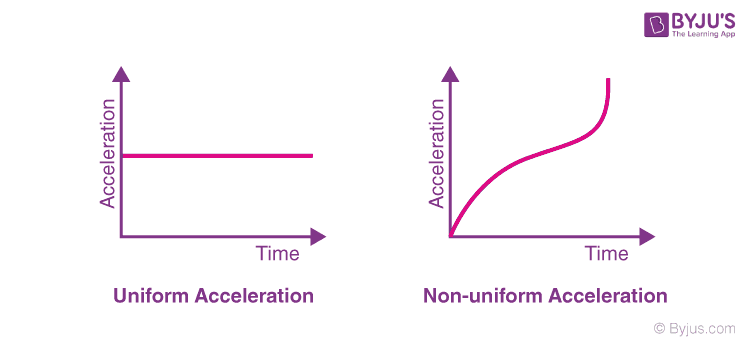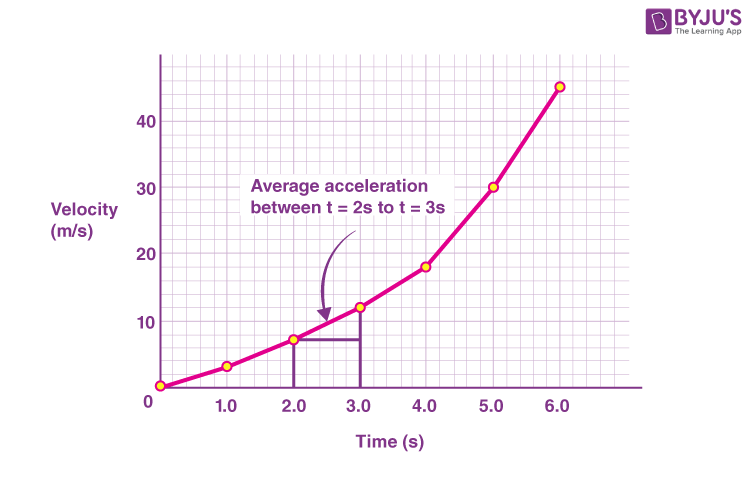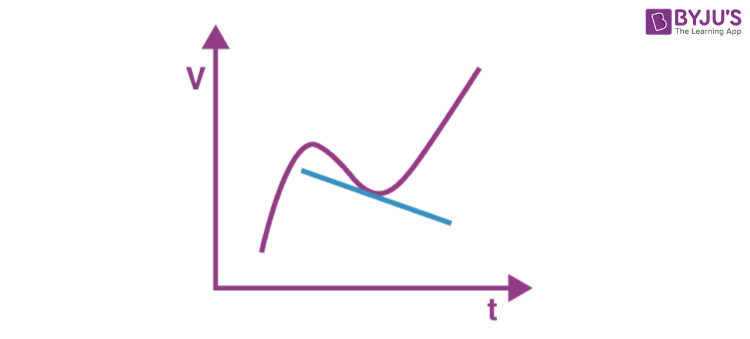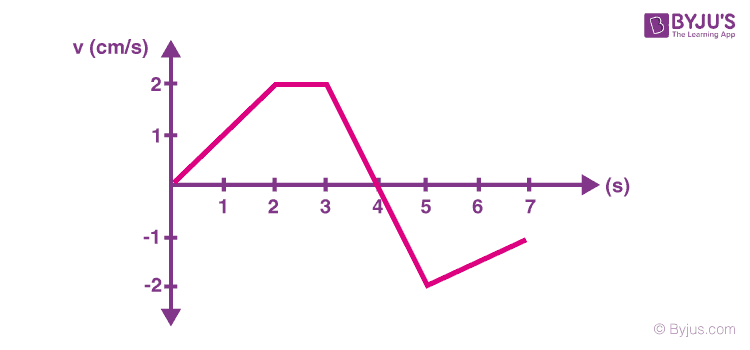# Acceleration

An object is said to be accelerated if there is a change in its velocity. The change in the velocity of an object could be an increase or decrease in speed or a change in the direction of motion. A few examples of acceleration are the falling of an apple, the moon orbiting around the earth, or when a car is stopped at the traffic lights. Through these examples, we can understand that when there is a change in the direction of a moving object or an increase or decrease in speed, acceleration occurs.

## What Is Acceleration?

Acceleration is defined as

The rate of change of velocity with respect to time.

Acceleration is a vector quantity as it has both magnitude and direction. It is also the second derivative of position with respect to time or it is the first derivative of velocity with respect to time.

## What Is the Acceleration Formula?

Acceleration formula is given as:

$$\begin{array}{l}acceleration = \frac{(final\;velocity)-(initial\;velocity)}{time}\end{array}$$
$$\begin{array}{l}acceleration = \frac{change\;in\;velocity}{time}\end{array}$$
$$\begin{array}{l}a= \frac{v_{f}-v_{i}}{t}\end{array}$$
 $$\begin{array}{l}a= \frac{\Delta v}{t}\end{array}$$

Where,

• a is the acceleration in m.s-2
• vis the final velocity in m.s-1
• vi is the initial velocity in m.s-1
• t is the time interval in s
• Δv is the small change in the velocity in m.s-1

## What Is the Unit of Acceleration?

The SI unit of acceleration is given as:

 SI unit m/s2

## Types of AccelerationRead More: SI unit of Acceleration

### Uniform and Non-uniform Acceleration

So can we have a situation when speed remains constant but the body is accelerated? Actually, it is possible in a circle where speed remains constant but since the direction is changing hence the velocity changes, and the body is said to be accelerated.

### Average Acceleration

The average acceleration over a period of time is defined as the total change in velocity in the given interval divided by the total time taken for the change. For a given interval of time, it is denoted as ā.

Mathematically,

$$\begin{array}{l} \overline{a} = \frac{ v _ { 2 } \, – \, v _ { 1 } }{ t _{ 2 } \, – \, t _{ 1 } } = \frac{ \Delta v }{ \Delta t } \end{array}$$

Where v2 and v1 are the instantaneous velocities at time t2 and t1 and ā is the average acceleration.

### What Is Instantaneous Acceleration?

Instantaneous acceleration is defined as

The ratio of change in velocity during a given time interval such that the time interval goes to zero.

Related Articles:

## Velocity-Time GraphAverage acceleration: In the velocity-time graph shown above, the slope of the line between the time interval t1 and t2 gives the average value for the rate of change of velocity for the object during the time t1 and t2.

Instantaneous acceleration: In a velocity-time curve, the instantaneous acceleration is given by the slope of the tangent on the v-t curve at any instant.### Positive, Negative and Zero AccelerationConsider the velocity-time graph shown above. Here, between the time intervals of 0-2 seconds, the velocity of the particle is increasing with respect to time; hence the body is experiencing a positive acceleration as the slope of the v-t curve in this time interval is positive.

Between the time intervals of 2-3 seconds, the velocity of the object is constant with respect to time; hence the body is experiencing zero acceleration as the slope of the v-t curve in this time interval is 0.

Now, between the time intervals of 3-5 seconds, the velocity of the body is decreasing with respect to time; hence the body experiences a negative value of the rate of change of velocity as the slope of the v-t curve in this time interval is negative.

## Click on the below Video to understand what is the velocity-time graph## Acceleration Example

Following are few solved examples of acceleration:

Q1. What will be the acceleration of an object which moves with uniform velocity?
Ans: Given,
The velocity is uniform, therefore the initial and final velocities are equal and is given as V.
∴From definition, acceleration is given as:

$$\begin{array}{l}a= \frac{v_{f}-v_{i}}{t}\end{array}$$
$$\begin{array}{l}a=\frac{0}{t}\end{array}$$
∴ a = 0

Q2. A truck is moving with a constant velocity, v = 5 m.s-1. The driver stops for diesel and the truck accelerates forward. After 20 seconds, the driver stops accelerating to maintain a constant velocity, v = 25 m.s-1. What is the truck’s acceleration?
Ans: Given,
Initial velocity, vi = 5 m.s-1
Final velocity, vf = 25 m.s-1
Time interval, t = 20 s
∴ By the definition, acceleration is given as:

$$\begin{array}{l}a= \frac{v_{f}-v_{i}}{t}\end{array}$$
$$\begin{array}{l}a=\frac{25-5}{20}\end{array}$$
∴ a=1 m.s-2

Q3. Find the final velocity of the ball that is dropped from the second floor if the ball takes 18 seconds before hitting the ground. The acceleration due to gravity is g = 9.80 m.s-2.
Ans: Given,
Initial velocity, vi = 0 m.s-1
Final velocity, vf = ?
Acceleration due to gravity = a = g = 9.80 m.s-2
Time interval, t = 18 s
∴ From the definition, acceleration is given as:

$$\begin{array}{l}a= \frac{v_{f}-v_{i}}{t}\end{array}$$
Rearranging the formula,
vf=vi + at
vf= 0+(9.80×18)

vf=176.4 m.s-1

### What Is the Difference between Acceleration and Velocity?

Following is the table of acceleration vs velocity:

 Parameter Acceleration Velocity Definition Acceleration is defined as the change in the velocity of an object with respect to time Velocity is defined as the speed of an object in a particular direction Formula Velocity/Time Displacement/Time Unit m.s-2 m.s-1

Related Article:

## Watch the video and learn about 2D motion and projectile motion## Frequently Asked Questions – FAQs

Q1

### What is centripetal acceleration?

When an object is moving in a circle and its acceleration vector is pointed towards the centre of that circle, it is known as centripetal acceleration. The unit of centripetal acceleration is m/s2.

Q2

### What is gravitational acceleration?

Gravitational acceleration is used for indicating the intensity of the gravitational field. It is expressed as m/s2 and its value on the surface of the earth is 9.8 m/s2.

Q3

### What is tangential acceleration?

Tangential acceleration is defined as the rate of change of the tangential velocity of a particle in a circular orbit. There are 3 possible values for tangential acceleration. First, when the magnitude of the velocity vector increases with time, tangential acceleration is greater than zero. Second, when the magnitude of the velocity vector decreases with time, tangential acceleration is less than zero. The third possibility is when the magnitude of the velocity vector remains constant, the tangential acceleration is equal to zero.

Q4

When there is an acceleration of an object along the radius directed towards the centre, it is known as radial acceleration. It is expressed as radian/sec2.

Q5

### What is Coriolis acceleration?

The acceleration due to the rotation of the earth is known as the Coriolis acceleration. This acceleration is experienced by the particles that move along the surface of the earth.